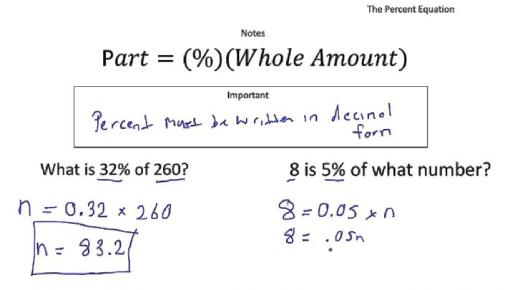# TABE: Quiz On Percent Equation! Math Trivia Questions

12 Questions | Attempts: 242
ShareSettingsWelcome to this amazing TABE quiz on Percent Equation Math Trivia Questions. Percentages represent a portion of something out of a whole which is represented by 100%, take up this challenging quiz and see if you can find the percentages of the numbers in each question. All the best and take your time choosing your best answer for each!

• 1.
190% of 53=
• 2.
90% of 62=
• 3.
132% of 90=
• 4.
30% of 70=
• 5.
30% of_=15
• 6.
100% of _=10
• 7.
40%  of _=20
• 8.
50%of_=10
• 9.
_%of 40=16
• 10.
_%of 40=36
• 11.
_% of 180=90
• 12.
_%of 140=70

## Related TopicsBack to top
×

Wait!
Here's an interesting quiz for you.